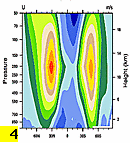cam6_3_015.clubb_F2000climo_ne30pg3_ne30pg3_mg17_1800pes_210404_3macmic_deepcunl_2yrs
and
cam6_3_015_F2000climo_ne30pg3_ne30pg3_mg17_1800pes_210401_3macmic_5yrs

DIAG Set 4 - Vertical contour plots of DJF, MAM, JJA, SON and ANN zonal means

Basic Variables DJF MAM JJA SON ANN
OMEGA * Pressure vertical velocity plotplotplotplotplot
QRL Longwave heating rate plotplotplotplotplot
QRS Shortwave heating rate plotplotplotplotplot
RELHUM * Relative humidity plotplotplotplotplot
SHUM * Specific humidity plotplotplotplotplot
T * Temperature plotplotplotplotplot
TDH * Total diabatic heating plotplotplotplotplot
TDM * Total diabatic moistening plotplotplotplotplot
U * Zonal wind plotplotplotplotplot
VQ Meridional moisture transport plotplotplotplotplot
VT Meridional heat transport plotplotplotplotplot
ZMMSF * Meridional stream function plotplotplotplotplot

Eddy Fluxes DJF MAM JJA SON ANN

EKE * transient eddy kinetic energy plotplotplotplotplot
OBSTAR*TBSTAR * stationary eddy vertical heat flux plotplotplotplotplot
OPTP BAR * transient eddy vertical heat flux plotplotplotplotplot
VBSTAR*QBSTAR * meridional stationary eddy moisture flux plotplotplotplotplot
VPQP BAR * meridional transient eddy moisture flux plotplotplotplotplot
VBSTAR*TBSTAR * meridional stationary eddy heat flux plotplotplotplotplot
VPTP BAR * meridional transient eddy heat flux plotplotplotplotplot
VBSTAR*UBSTAR * meridional stationary eddy momentum flux plotplotplotplotplot
VPUP BAR * meridional transient eddy momentum flux plotplotplotplotplot

Cloud Variables DJF MAM JJA SON ANN
CLOUD * cloud fraction plotplotplotplotplot
CWAT cloud water plotplotplotplotplot
CLDLIQ grid-box cloud liquid plotplotplotplotplot
CLDICE grid-box cloud ice plotplotplotplotplot
GCLDLWP ICE grid-box cloud ice water path plotplotplotplotplot
GCLDLWP LIQUID grid-box cloud liquid water path plotplotplotplotplot
ICLDTWP in-cloud ice+liquid water path plotplotplotplotplot
ICWMR in-cloud water mixing ratio (liquid) plotplotplotplotplot
ICIMR in-cloud water mixing ratio (ice) plotplotplotplotplot

*WGNE diagnostic variable

DIAG Set 4 - Vertical contour plots of DJF, MAM, JJA, SON and ANN zonal means

Basic Variables DJF MAM JJA SON ANN
OMEGA * Pressure vertical velocity plotplotplotplotplot
QRL Longwave heating rate plotplotplotplotplot
QRS Shortwave heating rate plotplotplotplotplot
RELHUM * Relative humidity plotplotplotplotplot
SHUM * Specific humidity plotplotplotplotplot
T * Temperature plotplotplotplotplot
TDH * Total diabatic heating plotplotplotplotplot
TDM * Total diabatic moistening plotplotplotplotplot
U * Zonal wind plotplotplotplotplot
VQ Meridional moisture transport plotplotplotplotplot
VT Meridional heat transport plotplotplotplotplot
ZMMSF * Meridional stream function plotplotplotplotplot

Eddy Fluxes DJF MAM JJA SON ANN
EKE * transient eddy kinetic energy plotplotplotplotplot
OBSTAR*TBSTAR * stationary eddy vertical heat flux plotplotplotplotplot
OPTP BAR * transient eddy vertical heat flux plotplotplotplotplot
VBSTAR*QBSTAR * meridional stationary eddy moisture flux plotplotplotplotplot
VPQP BAR * meridional transient eddy moisture flux plotplotplotplotplot
VBSTAR*TBSTAR * meridional stationary eddy heat flux plotplotplotplotplot
VPTP BAR * meridional transient eddy heat flux plotplotplotplotplot
VBSTAR*UBSTAR * meridional stationary eddy momentum flux plotplotplotplotplot
VPUP BAR * meridional transient eddy momentum flux plotplotplotplotplot

Cloud Variables DJF MAM JJA SON ANN
CLOUD * cloud fraction plotplotplotplotplot
CWAT grid-box cloud water plotplotplotplotplot
CLDLIQ grid-box cloud liquid plotplotplotplotplot
CLDICE grid-box cloud ice plotplotplotplotplot
GCLDLWP ICE grid-box cloud ice water path plotplotplotplotplot
GCLDLWP LIQUID grid-box cloud liquid water path plotplotplotplotplot
ICLDTWP in-cloud ice+liquid water path plotplotplotplotplot
ICWMR in-cloud water mixing ratio (liquid) plotplotplotplotplot
ICIMR in-cloud water mixing ratio (ice) plotplotplotplotplot

Microphysics Variables DJF MAM JJA SON ANN
SIWC Snow + Ice Water Content plotplotplotplotplot
WSUB Sub-grid Scale Vertical Velocity plotplotplotplotplot
CCN3 Cloud Condensation Nuclei plotplotplotplotplot
AWNC Avg. Cloud Water Number Concentration plotplotplotplotplot
AWNI Avg. Cloud Ice Number Concentration plotplotplotplotplot
AREL Avg. Droplet Effective Radius plotplotplotplotplot
AREI Avg. Ice Effective Radius plotplotplotplotplot
AQRAIN Avg. Rain Mixing Ratio plotplotplotplotplot
AQSNOW Avg. Snow Mixing Ratio plotplotplotplotplot
ANRAIN Avg. Rain Number Concentration plotplotplotplotplot
ANSNOW Avg. Snow Number Concentration plotplotplotplotplot

CFMIP Observation Simulator Package (COSP)

CLOUDSAT-COSP From Level 2 GEOPROF Jun2006-Nov2010 DJF MAM JJA SON ANN
CFAD_DBZE94_CS Hydrometeor occurrence (dBZe > -25) (COSP) plotplotplotplotplot

*WGNE diagnostic variable